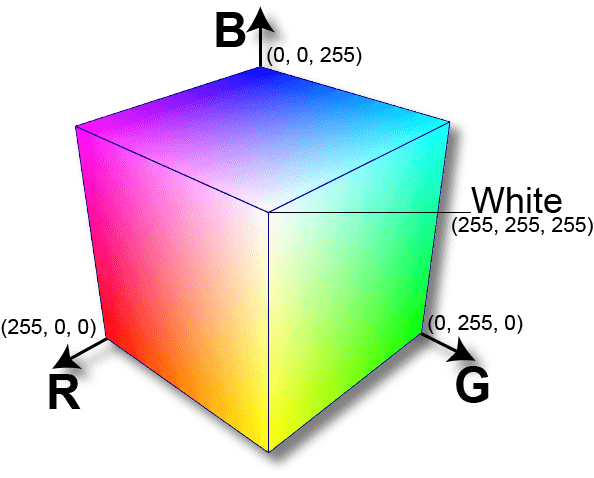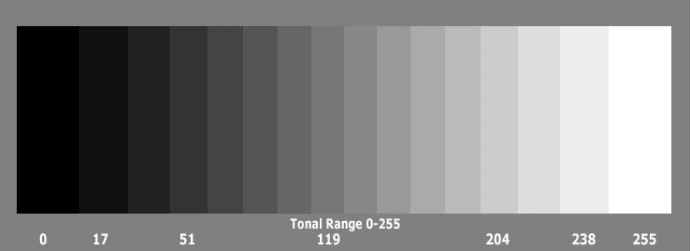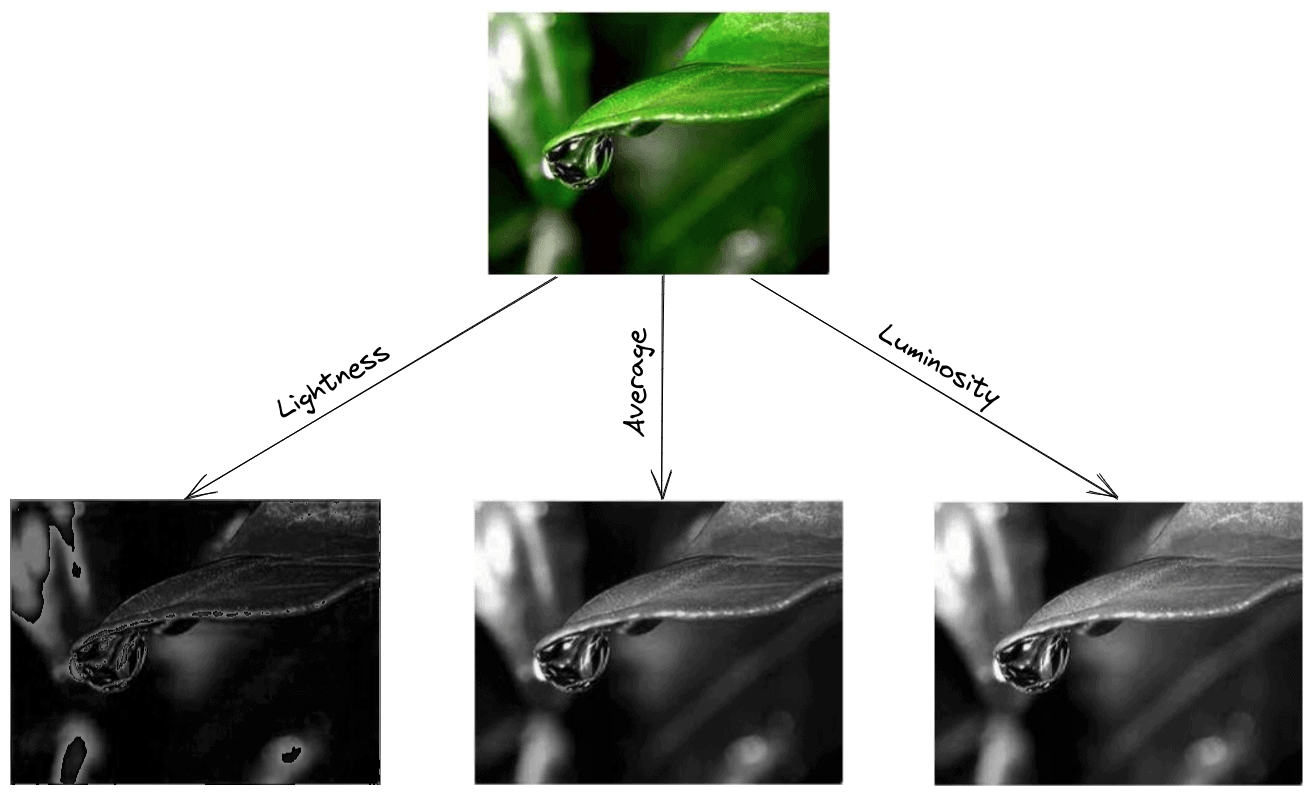## 1. Overview

In this tutorial, we’ll talk about how we can convert an RGB image to grayscale. First, we’ll make a brief introduction to the color models, and then we’ll present three conversion methods along with an example.

## 2. Color Models

Representing colors as numerical values is a necessary step in many applications. To do this, we use models that are mathematical models that describe ways of mapping colors to a set of numbers. Usually, a color model defines three or four color components that are easily described through a coordinate system. Each color that the model can represent corresponds to a point in this coordinate system.

Along with these values, those models also provide a description of how to interpret these components in order to generate a color.

### 2.1. RGB

The most well-known color model is RGB which stands for Red-Green-Blue. As the name suggests, this model represents colors using individual values for red, green, and blue. The RGB model is used in almost all digital screens throughout the world.

Specifically, a color is defined using three integer values from 0 to 255 for red, green, and blue, where a zero value means dark and a value of 255 means bright. Given the values, the final color is defined when we mix these three basic colors weighted by their values.

If we mix the three colors equally (RGB = (255, 255, 255)), we’ll get white while the absence of all colors (RGB = (0, 0, 0)) means black. Below there is the RGB coordinate system where we can see all the different colors that the model can describe:### 2.2. Grayscale

Grayscale is the simplest model since it defines colors using only one component that is lightness. The amount of lightness is described using a value ranging from 0 (black) to 255 (white).

On the one hand, grayscale images convey less information than RGB. However, they are common in image processing because using a grayscale image requires less available space and is faster, especially when we deal with complex computations.

Below, we can see the full range of colors that the grayscale model can describe:### 2.3. Others

Depending on the use case, there have been numerous proposed models throughout the years. For example, the CMYK model is used in printing and describes colors using values for cyan, magenta, yellow and black. HSL and HSV are two colors used by artists since they’re more aligned with the way human vision perceives a color. The basic components are hue, saturation, and lightness or value, respectively.

## 3. Convert RGB to Grayscale

Now, let’s talk about the main goal of this tutorial which is how to convert an image from RGB to grayscale. Since these models are based on different interpretations of color perception (primary colors and lightness), there is not a perfect way for conversion. There are some commonly used methods like the ones below.

### 3.1. Lightness Method

A very simple method is to take the average value of the components with the highest and lowest value:We can easily see that this method presents a very serious weakness since one RGB component is not used. This is definitely a problem because the amount of lightness that our eye perceives depends on all three basic colors.

### 3.2. Average Method

Another method is to take the average value of the three components (red, green, and blue) as the grayscale value:Although we now take into account all components, the average method is also problematic since it assigns the same weight to each component. Based on research on human vision, we know that our eyes react to each color in a different manner. Specifically, our eyes are more sensitive to green, then to red, and finally to blue. Therefore, the weights in the above equation should change.

### 3.3. Luminosity Method

The best method is the luminosity method that successfully solves the problems of previous methods.

Based on the aforementioned observations, we should take a weighted average of the components. The contribution of blue to the final value should decrease, and the contribution of green should increase. After some experiments and more in-depth analysis, researchers have concluded in the equation below:## 4. Example

Finally, let’s apply these three methods in an image to further illustrate our results. Below, we can see the results of applying the lightness, the average, and the luminosity method to convert an RGB image to grayscale:As expected, the lightness method is the worst since it completely fails to capture the lightness of the input image. Among the other two, luminosity is the best since the average method produces a darker grayscale image.

## 5. Conclusion

In this tutorial, we discussed how we could convert an RGB image to grayscale. First, we talked about the different color models, and then we presented three conversion methods along with an example.